FAQ's
• A digital transistor is a bipolar transistor that integrates resistors.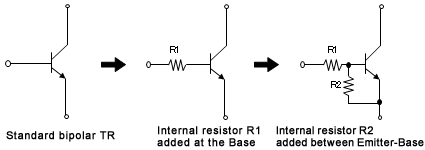¦Concerning internal resistor R1

• The role of R1 is to stabilize transistor operation by converting the input voltage to current.

Bipolar operation tends to become unstable if the input (Base) is connected directly to the output terminal of an IC for voltage control. Inserting a resistor at the Base stabilizes operation. Please note that the output current will vary exponentially based on changes to the input voltage, but will maintain a linear relationship with the input current.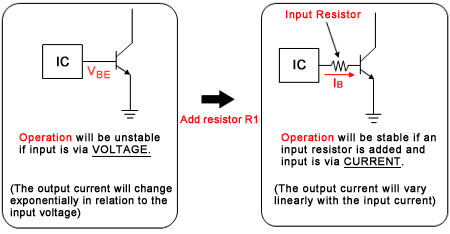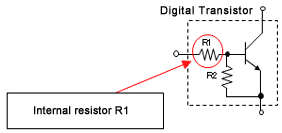Next we compare the difference between Voltage and Current control.
 Voltage ControlInput : Emitter-Base Voltage VEB Current ControlInput : Base Current IB Measurement Circuit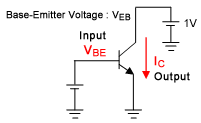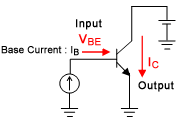Theoretical Formula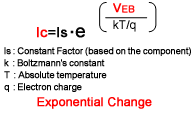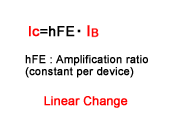Input-Output Characteristics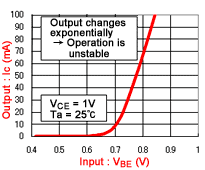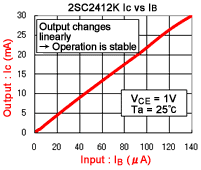As we can see in the above graphs, Voltage Control results in an exponential change in the output current based on the input voltage, while Current Control exhibits a linear relationship between the input and output currents.
For example, in the Current Control graph at right a doubling of the input current, from 40uA to 80uA, results in twice the output current, from 9mA to 18mA. However, in the Voltage Control graph we see that a small change in the input voltage, from 0.7V to 0.8V, results in an increase in the output current by 7 times, from 10mA to 70mA. This is not desirable, since even a little noise introduced in the input voltage can result in significant changes to the output current.
Thus it is evident that current control via Base resistor that converts the voltage into current results in stable drive. ROHM offers digital transistors, which integrate a resistor at the Base, reducing the number of external components and mounting area.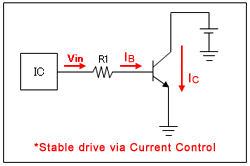¦Regarding internal resistor R2

• The role of R2 is to absorb leakage current and shunt it to ground in order to prevent malfunction.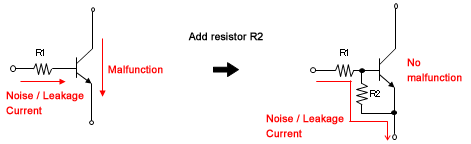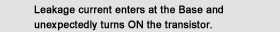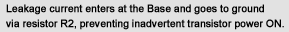Please note that a large input current may cause the transistor to turn ON,regardless of the presence of resistor R2.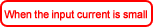All of the current flows to ground via R2, preventing TR power ON(and possible malfunction in the case of leakage current).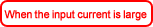Part of the current flows through the Base, causing the transistor to turn ON(inadvertently in the case of leakage current).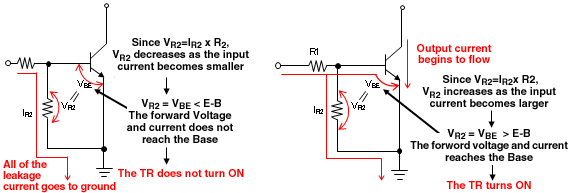VR2=VBE<(when E-B Forward Voltage ? 0.7V) VR2=VBE>(when E-B Forward Voltage ? 0.7V)
• Products: Digital Transistors